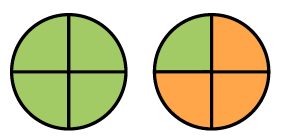### Home > ACC7 > Chapter 9 Unit 6 > Lesson CC2: 9.1.2 > Problem9-37

9-37.

Jessica’s parents are planning their new house. They received a scale drawing from an architect, and Jessica is trying to figure out how big her bedroom will be. The architect has noted that each $\frac { 1 } { 4 }$ inch on his drawing represents one foot of distance in the new house. If the diagram of Jessica’s room measures $2\frac{1}{2}$ inches by $4\frac{1}{4}$ inches, what will be the actual dimensions of her new room?

How many $\frac { 1 } { 4 }$ inches are there in $2\frac{1}{2}$ and $4\frac{1}{4}$ inch measurements?

For example, say Jessica's bathroom is represented as a rectangle measuring $1$ and one quarter inches by $2$ inches. Using the same scale, you can find the actual dimensions of her new bathroom.

The diagram below visually shows that there are $5$ one fourths in one and one fourth inches. Now draw the same thing for two inches.There are $5$ one fourths in one and one fourth and $8$ one fourths in two.

Now you can use the distance conversion and multiplication to find the actual dimensions of the bathroom. Since one-fourth inch is equivalent to 1 foot, Jessica's bathroom will be $5$ feet by $8$ feet.

Can you use a similar strategy to find the dimensions of Jessica's bedroom? Drawing a diagram may help!

Jessica's bedroom will be $10$ feet by $17$ feet.